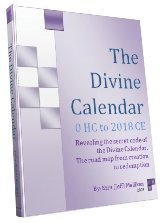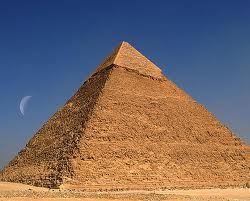Select Page

It all started with the 42 Letter Name of G-d. And it’s all supposed to end (shift) in 5778HC.
The 42 Letters are officially and primordially represented by and  guided by the energy of the Hebrew letters Mem-Bet (MB) of numerical value 42. The ordinal value, or placement value within the Hebrew alphabet, of these two letters is 13 for Mem and 2 for Bet, which are the 13th and the 2nd letters of the Hebrew alphabet and together are 15.  Thus the complete value of the Mem-Bet (MB) is 42 plus 15, or 57.
The number 42 is always represent by Mem-Bet (MB) because that is the simplest way to write 42 in Hebrew and it is how they count in Hebrew and always has been. Likewise,  57 is represented by the two letters Nun-Zayin (NTz), which individually have values of 50 and 7 respectively, and ordinal values of 14 and 7 respectively. When we sum their values and their ordinal values together, we get a complete value of 57 + 21 = 78.Thus we go from 42 in the beginning to 57-78 in the end. Proportionately 42 is to 57 as 57 is to 78, all about 73% of one another.  While 73 is the numerical value of the word chochma in Hebrew, meaning both Wisdom and the 2nd highest sefira (dimension) to G-d, we know that the first verse of the Torah, the one from which the 42-Letter Name of G-d is derived, has a total numerical value equivalent to the sum of all the integers through 73.  This is Wisdom.  It is Light, and 42 x 57 x 78 is very close to the speed of Light,
There are many reason the year 5778 (2018 CE) was chosen as the date for the final redemption and Moshiach (the messiah) and we’ve written about many of them. The one given above isn’t much of a reason, as it is just another example of an increasingly more obvious divine plan that incorporates the secrets of the Torah, the Hebrew alphabet, and our base-10 math system. The Wisdom glows wherever we shine our flashlights, all we need to do is switch them on.
Illuminating the digits in  42, 57, and 78 we get 4+2+5+7+7+8 = 33 and 42 +57 +78 +33 = 210, as in the 210 years the Israelites where in exile in Egypt, and as in the height of the Great Pyramid in Giza in both number of levels (210) and mir cubits (210). That Pyramid, which is also call Joseph’s Pyramid is just about 5778 inches high.
And if you are fond of the divine beauty in design, please note that the proportion of 33 to 42 or 33/42 is .7857142, and unscrambling the 3 double-digit numbers we began this discussion with, we get 1, 42, 57, and 78.  G-d is One.
L’ag b’omer is the 33rd day of the Omer, let’s all connect that day to Rabbi Shimon, the author of the Zohar and to the energy of Moshiach consciousness.